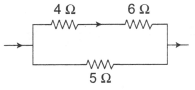In the circuit shown in figure, the heat produced in the 5  Ω resistor due to the current flowing through it is 10 cals/s.  The heat generated in the 4  Ω resistor is

# In the circuit shown in figure, the heat produced in the $5\text{\hspace{0.17em}\hspace{0.17em}}\Omega$ resistor due to the current flowing through it is 10 cals/s.  The heat generated in the $4\text{\hspace{0.17em}\hspace{0.17em}}\Omega$ resistor is1. A

1 cal/s

2. B

2 cal/s

3. C

3 cal/s

4. D

4 cal/s

Fill Out the Form for Expert Academic Guidance!l

+91

Live ClassesBooksTest SeriesSelf Learning

Verify OTP Code (required)

### Solution:

$\begin{array}{l}{\mathrm{P}}_{5}={\mathrm{i}}_{5}^{2}\mathrm{R},\text{\hspace{0.17em}\hspace{0.17em}\hspace{0.17em}}{\mathrm{P}}_{5}=\left({\mathrm{i}}_{5}^{2}\right)5\\ {\mathrm{i}}_{4}=\frac{{\mathrm{i}}_{5}}{2}\text{\hspace{0.17em}}⇒\text{\hspace{0.17em}\hspace{0.17em}}{\mathrm{P}}_{4}\text{\hspace{0.17em}}{\left(\frac{{\mathrm{i}}_{5}}{2}\right)}^{2}\text{\hspace{0.17em}\hspace{0.17em}}×\text{\hspace{0.17em}\hspace{0.17em}}4\\ \frac{{\mathrm{P}}_{4}}{{\mathrm{P}}_{5}}=\frac{1}{5}\text{\hspace{0.17em}}⇒\text{\hspace{0.17em}\hspace{0.17em}}{\mathrm{P}}_{4}=\frac{{\mathrm{P}}_{5}}{5},\text{\hspace{0.17em}\hspace{0.17em}\hspace{0.17em}\hspace{0.17em}}{\mathrm{P}}_{4}=\frac{10}{4}\text{\hspace{0.17em}\hspace{0.17em}}=\text{\hspace{0.17em}\hspace{0.17em}}2\mathrm{cal}/\mathrm{s}\end{array}$

## Related content

 Karnataka Traditional Dress and Culture Entrance Examinations in India Maharashtra Traditional Dress for Men and Women My Daily Routine Paragraph In English Environment Pollution Paragraph for Students 100, 150, 200, 250 & 300 words Past Perfect Continuous Tense Patriotic Slogans by Freedom Fighters of India GK Questions and Answers on Solar System Past Perfect Tense Healthy Food Slogans+91

Live ClassesBooksTest SeriesSelf Learning

Verify OTP Code (required)